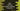# 7 different ways to find the largest, smallest number in dart list# Introduction :

In Dart, we have a couple of different ways to find out the maximum and minimum value in a list. We can iterate through a list or use other list methods to find the maximum/minimum.

In this post, I will show you 7 different ways to find out the maximum and minimum list value.

## Method 1: using a for loop :

We can iterate through a list using any loop.

• Initialize two variables for holding the largest and smallest numbers of the list. - Assign the first element of the list to both of these variables.
• Iterate through the list elements one by one and compare each element of the list with the smallest and largest number variables. Update these variables accordingly.

I am using one for loop in this example, but you can also use a while loop if you want.

``````main() {
var given_list = [122, 12, 33, 14, 5];
var largest_value = given_list;
var smallest_value = given_list;

for (var i = 0; i < given_list.length; i++) {
if (given_list[i] > largest_value) {
largest_value = given_list[i];
}
if (given_list[i] < smallest_value) {
smallest_value = given_list[i];
}
}

print("Smallest value in the list : \${smallest_value}");
print("Largest value in the list : \${largest_value}");
}``````

It will print the below output :

``````Smallest value in the list : 5
Largest value in the list : 122``````

## Method 2: Sorting the list :

Not an efficient method, but we can always sort a list and pick the first and the last element of that list.

Dart list comes with a method called sort() that can be used for sorting. This method sorts a list in a natural order. So, the first element is the smallest and the last element is the largest for a sorted list.

``````main() {
var given_list = [122, 12, 33, 14, 5];
given_list.sort();

print(given_list.first);
print(given_list.last);
}``````

## Method 3: Using forEach :

The forEach method is used to iterate over the list elements one by one. Similar to the for loop example, we can initialize two variables to hold the largest and the smallest number in the list and update these variables while iterating.

``````main() {
var given_list = [122, 12, 33, 14, 5, 555];
var largest_value = given_list;
var smallest_value = given_list;

given_list.forEach((e) => {
if (e > largest_value) {largest_value = e},
if (e < smallest_value) {smallest_value = e},
});

print("Smallest value in the list : \${smallest_value}");
print("Largest value in the list : \${largest_value}");
}``````

It will print :

``````Smallest value in the list : 5
Largest value in the list : 555``````

## Method 4: Using reduce :

reduce method is used to reduce a list to a single value. It takes one function and reduce the list using that function. We can provide a function to reduce a list to the largest and the smallest element of a list.

``````main() {
var given_list = [122, 12, 33, 14, 5, 555];

var smallest_value =
given_list.reduce((current, next) => current < next ? current : next);
var largest_value =
given_list.reduce((current, next) => current > next ? current : next);

print("Smallest value in the list : \${smallest_value}");
print("Largest value in the list : \${largest_value}");
}``````

## Method 5: Using dart:math and reduce :

We can also use the max and min functions of dart:math library with reduce to find out the maximum and minimum value in a list.

``````import "dart:math";

main() {
var given_list = [122, 12, 33, 14, 5, 555];

var smallest_value = given_list.reduce(min);
var largest_value = given_list.reduce(max);

print("Smallest value in the list : \${smallest_value}");
print("Largest value in the list : \${largest_value}");
}``````

Simple and clean.

## Method 6: Using fold :

fold is similar to reduce. It takes one initial value and reduces the list to a single value by combining each element of the list with the existing initial value. We will pass the first element of the list as the initial value :

``````main() {
var given_list = [122, 12, 33, 14, 5, 555];

var smallest_value = given_list.fold(given_list,
(int previous, int current) => previous < current ? previous : current);
var largest_value = given_list.fold(given_list,
(int previous, int current) => previous > current ? previous : current);

print("Smallest value in the list : \${smallest_value}");
print("Largest value in the list : \${largest_value}");
}``````

## Method 7: Using dart:math and fold :

Similar to reduce, we can also use dart:math library with fold :

``````import "dart:math";

main() {
var given_list = [122, 12, 33, 14, 5, 555];

var smallest_value = given_list.fold(given_list, min);
var largest_value = given_list.fold(given_list, max);

print("Smallest value in the list : \${smallest_value}");
print("Largest value in the list : \${largest_value}");
}``````